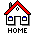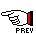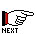Introduction

A typical engineering problem involves a network of system components that can be modeled mathematically with a set of linear equations established with certain physical laws. The unknowns in the equations represent the properties that the engineer would like to investigate, given a set of known conditions. In this example, we use chemical reactors that mix conservative chemical fluid from input sources with various concentrations and dispense the new mixture to other mixers and/or sinks. A pipe used to link two components contains the flow rate of the mixture that travels from one component to the other. Normally, the sum of all the input flow rates is equal to the sum of all the output flow rates in a mixer so that the volume can stay constant. The concentration in each mixer (assumed to be uniform) are the unknowns that we would like to solve. Applying the law of mass conservation, we can set up a system of linear equations according to the configuration of the mixers, the sources, and the sinks with their concentrations and the pipes with their flow rates. The left-hand-side (l-h-s) constants of the equations are calculated and stored in a matrix while the right-hand-side constants are stored in a table. The table also includes memory spaces for the solution vector.

With the SansGUI Modeling and Simulation Environment, the simulator is implemented in an object-oriented manner. Using the SansGUI Run-Time Environment, instances of mixers, sources and sinks can be created and connected with pipes, derived from the Link class. The linear equation solver is implemented in Class Solver, derived from Class Collection. Three calculation matrices and a reactor table are reference objects used during the calculation process. Three solution methods are available from the simulator: 1) using Naive Gauss Elimination, 2) using LU Decomposition, and 3) calling MATLAB engine. We implemented the algorithms found in Chapra and Canale [Cha98] for the first two methods in C/C++ and Fortran independently. The third method requires the MATLAB software from the Mathworks, Inc. This example is one of our answers to many inquiries about how to use SansGUI in conjunction with the MATLAB engine. Again, both C/C++ and Fortran implementation of the MATLAB engine call are provided. Except for the Naive Gauss Elimination method, an inverse matrix of the original constant matrix can be obtained for further understanding of the properties of a mixer network.

## ContentsMixer Example for SansGUI Version 1.0